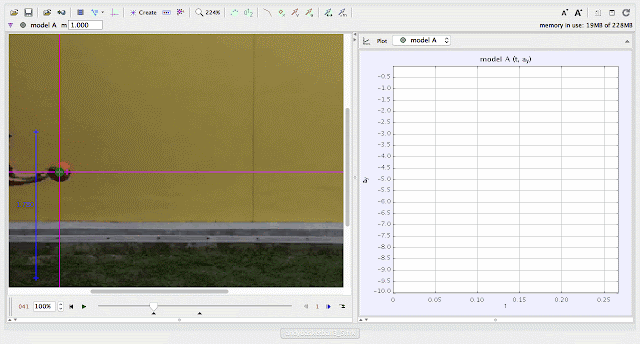{source}
<?phprequire_once JPATH_SITE.'/TTcustom/TT_contentparser.php';//include 'TT_contentparser.php';$$parameters = array( "topicname" => "02_newtonianmechanics_2kinematics", //e.g. 02_newtonianmechanics_2kinematics"modelname" => "andybasketball3_5"); //e.g. airpuckconstantvelocity.trzecho generateSimHTML($$parameters, "TRZ");
?>
{/source}

### ICT Connection Lessonusing Tracker Model Builder to create theoretic graphs for simplifying Physics concepts. projectile motion is illustrated through displacement sy versus time graphusing Tracker Model Builder to create theoretic graphs for simplifying Physics concepts. projectile motion is illustrated through velocity vy versus time graphusing Tracker Model Builder to create theoretic graphs for simplifying Physics concepts. projectile motion is illustrated through acceleration ay versus time graph

end faq

4.5 1 1 1 1 1 1 1 1 1 1 Rating 4.50 (1 Vote)